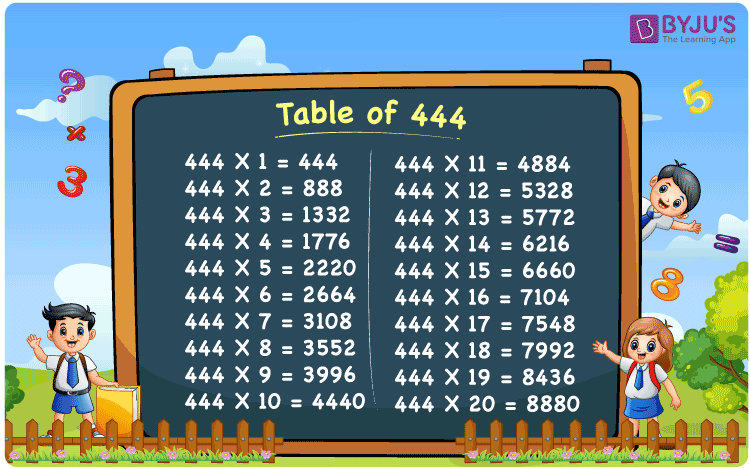Checkout JEE MAINS 2022 Question Paper Analysis : Checkout JEE MAINS 2022 Question Paper Analysis :

# Table of 444

The table of 444 is a multiplication table formed by multiplying 444 with natural numbers. It is helpful to learn the 444 times table because it speeds up the completion of mathematical problems. In this page, we have provided a 444 times table chart as well as a tabular version of the multiplication table of 444. By clicking the link below, you can download the 444 table in PDF format.

## Table of 444 Chart## What is the 444 Times Table?

The table of 444 is obtained using the repeated addition of 444 for “n” number of times, where “n” represents the natural number.

For example, 444 × 3 = 1332, which is the same as 444 + 444 + 444 = 1332.

The below table displays the multiplication and the repeated addition of 444.

 444×1 = 444 444 444×2 = 888 444 + 444 = 888 444×3 = 1332 444 + 444 + 444 = 1332 444×4 = 1776 444 + 444 + 444 + 444 = 1776 444×5 = 2220 444 + 444 + 444 + 444 + 444 = 2220 444×6 = 2664 444 + 444 + 444 + 444 + 444 + 444 = 2664 444×7 = 3108 444 + 444 + 444 + 444 + 444 + 444 + 444 = 3108 444×8 = 3552 444 + 444 + 444 + 444 + 444 + 444 + 444 + 444 = 3552 444×9 = 3996 444 + 444 + 444 + 444 + 444 + 444 + 444 + 444 + 444 = 3996 444×10 = 4440 444 + 444 + 444 + 444 + 444 + 444 + 444 + 444 + 444 + 444 = 4440

## Multiplication Table of 444

Go through the table of 444 presented in the tabular form.

 444 × 1 = 444 444 × 2 = 888 444 × 3 = 1332 444 × 4 = 1776 444 × 5 = 2220 444 × 6 = 2664 444 × 7 = 3108 444 × 8 = 3552 444 × 9 = 3996 444 × 10 = 4440 444 × 11 = 4884 444 × 12 = 5328 444 × 13 = 5772 444 × 14 = 6216 444 × 15 = 6660 444 × 16 = 7104 444 × 17 = 7548 444 × 18 = 7992 444 × 19 = 8436 444 × 20 = 8880

## Solved Example on the Table of 444

Example:

Determine the value of “k”, if 444k = 4884.

Solution:

Given that, 444k = 4884.

Thus, k = 4884/444

k = 11

Hence, the value of k is 11.

## Frequently Asked Questions on the Table of 444

### What is the table of 444?

444×1 = 444, 444×2 = 888, 444×3 = 1332, 444×4 = 1776, 444×5 = 2220, 444×6 = 2664, 444×7 = 3108, 444×8 = 3552, 444×9 = 3996, 444×10 = 4440.

### How many times does 444 equal 2664?

6 times 444 equals 2664.

### What is 444 times 20?

444 times 20 is 8880. I.e., 444 × 20 = 8880.